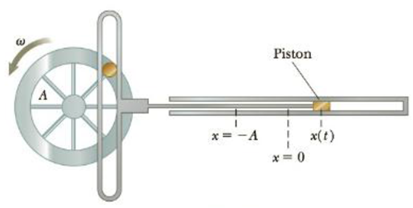Chapter 13, Problem 23P

Chapter
Section
Textbook Problem

The wheel in the simplified engine of Figure P13.23 has radius A = 0.250 m and rotates with angular frequency ω = 12.0 rad/s. At t = 0, the piston is located at x = A. Calculate the piston’s (a) position, (b) velocity, and (c) acceleration at t = 1.15 s.Figure P13.23

a)

To determine
The position of the piston.

Explanation

Given info: The radius of the wheel A=0.250m . The wheel rotates with angular frequency ω=12.0rads-1 . At t=0 , the piston is located at x=A . t=1.15s .

Explanation:

Since the piston is connected to the rotating wheel, its position is given by,

x=Asin(ωt)

Here,

x is the position of the piston

A is the radius of the reference circle

ω is the angular speed

t is the time taken

Substituting ω=12.0rads-1 , A=0

b)

To determine
The velocity of the piston.

c)

To determine
The acceleration of the piston.

Still sussing out bartleby?

Check out a sample textbook solution.

See a sample solution

The Solution to Your Study Problems

Bartleby provides explanations to thousands of textbook problems written by our experts, many with advanced degrees!

Get Started

What is the difference between H and E?

Chemistry: An Atoms First Approach

How many autosomes are present in a body cell of a human being? In a gamete?

Human Heredity: Principles and Issues (MindTap Course List)

3.30 Define the term spectator ion.

Chemistry for Engineering Students

What is a joint?

Human Biology (MindTap Course List)